# Transformations

In this lesson, we will determine the different types of Transformations that can be applied to the parent function $$f(x) = x^2$$ by expressing it in the Vertex Form $$f(x) = a(x-h)^2 + k$$.

## Reflection, Stretching and Compression

The $$a$$ value will both determine whether the quadratic function will get either stretched or compressed and which direction it will open:
• If $$|a| > 1$$, the graph will get stretched by a factor of $$a$$. This will make the graph appear narrower, as the x value will have a greater effect on the y value
• If $$|a| < 1$$, the graph will get compressed by a factor of $$a$$. This will make the graph appear wider, as the x value won't have as greate of an effect on the y value
• If $$a > 0$$, the function will be positive, making the parabola open upward
• If $$a < 0$$, the function will be negative, reflecting the graph and making the parabola open downward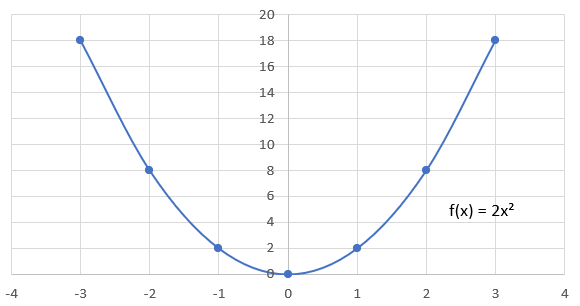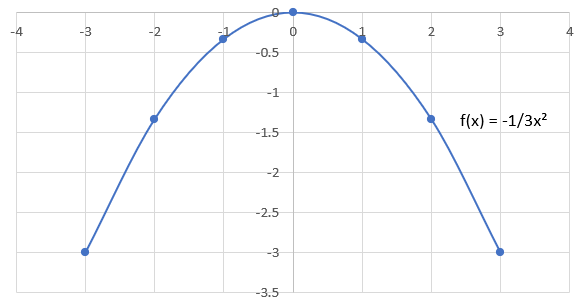State the transformation(s) in the quadratic equation $$f(x) = -6x^2$$

## Horizontal Shifts

The $$h$$ value will determine which direction horizontally the graph will get shifted. This change can be represented algebraically by the equation $$f(x) = (x-h)^2$$:
• If $$h > 0$$, the graph will get shifted to the right by $$h$$ units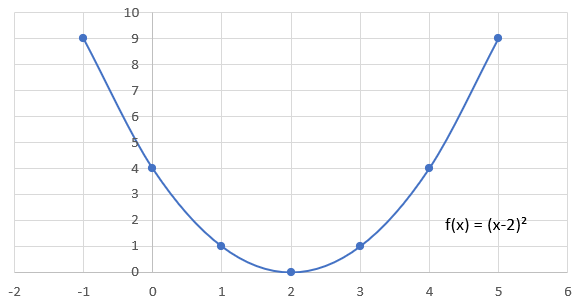• If $$h < 0$$, the graph will get shifted to the left by $$h$$ units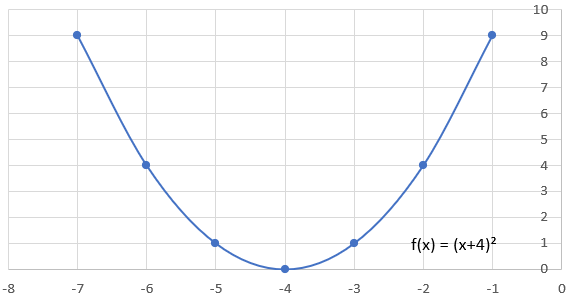Identify the quadratic equation for a graph that is shifted $$5$$ units left

## Vertical Shifts

The $$k$$ value will determine which direction vertically the graph will get shifted. This change can be represented algebraically by the equation $$f(x) = x^2 + k$$:
• If $$k > 0$$, the graph will get shifted upwards $$k$$ units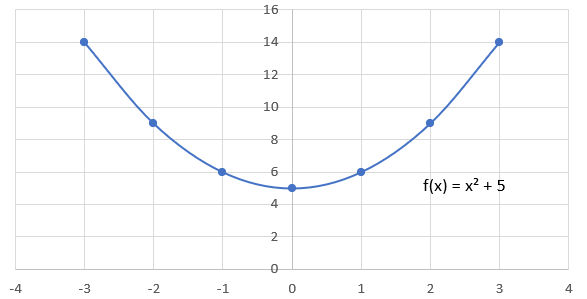• If $$k < 0$$, the graph will get shifted downwards $$k$$ units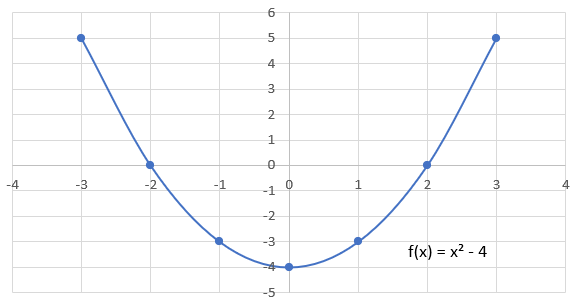Identify the quadratic equation for a graph that is shifted $$7$$ units upward

Identify and graph a qudratic equation that is reflected, stretched by a factor of $$4$$, shifted $$3$$ units right and shifted $$2$$ units downward